# Benchmarking Nearest Neighbor Searches in Python

I recently submitted a scikit-learn pull request containing a brand new ball tree and kd-tree for fast nearest neighbor searches in python. In this post I want to highlight some of the features of the new ball tree and kd-tree code that's part of this pull request, compare it to what's available in the scipy.spatial.cKDTree implementation, and run a few benchmarks showing the performance of these methods on various data sets.

My first-ever open source contribution was a C++ Ball Tree code, with a SWIG python wrapper, that I submitted to scikit-learn. A Ball Tree is a data structure that can be used for fast high-dimensional nearest-neighbor searches: I'd written it for some work I was doing on nonlinear dimensionality reduction of astronomical data (work that eventually led to these two papers), and thought that it might find a good home in the scikit-learn project, which Gael and others had just begun to bring out of hibernation.

After a short time, it became clear that the C++ code was not performing as well as it could be. I spent a bit of time writing a Cython adaptation of the Ball Tree, which is what currently resides in the sklearn.neighbors module. Though this implementation is fairly fast, it still has several weaknesses:

• It only works with a Minkowski distance metric (of which Euclidean is a special case). In general, a ball tree can be written to handle any true metric (i.e. one which obeys the triangle inequality).
• It implements only the single-tree approach, not the potentially faster dual-tree approach in which a ball tree is constructed for both the training and query sets.
• It implements only nearest-neighbors queries, and not any of the other tasks that a ball tree can help optimize: e.g. kernel density estimation, N-point correlation function calculations, and other so-called Generalized N-body Problems.

I had started running into these limits when creating astronomical data analysis examples for astroML, the Python library for Astronomy and Machine Learning Python that I released last fall. I'd been thinking about it for a while, and finally decided it was time to invest the effort into updating and enhancing the Ball Tree. It took me longer than I planned (in fact, some of my first posts on this blog last August came out of the benchmarking experiments aimed at this task), but just a couple weeks ago I finally got things working and submitted a pull request to scikit-learn with the new code.

## Features of the New Ball Tree and KD Tree¶

The new code is actually more than simply a new ball tree: it's written as a generic N dimensional binary search tree, with specific methods added to implement a ball tree and a kd-tree on top of the same core functionality. The new trees have a lot of very interesting and powerful features:

• The ball tree works with any of the following distance metrics, which match those found in the module scipy.spatial.distance:

['euclidean', 'minkowski', 'manhattan', 'chebyshev', 'seuclidean', 'mahalanobis', 'wminkowski', 'hamming', 'canberra', 'braycurtis', 'matching', 'jaccard', 'dice', 'kulsinski', 'rogerstanimoto', 'russellrao', 'sokalmichener', 'sokalsneath', 'haversine']

Alternatively, the user can specify a callable Python function to act as the distance metric. While this will be quite a bit slower than using one of the optimized metrics above, it adds nice flexibility.

• The kd-tree works with only the first four of the above metrics. This limitation is primarily because the distance bounds are less efficiently calculated for metrics which are not axis-aligned.

• Both the ball tree and kd-tree implement k-neighbor and bounded neighbor searches, and can use either a single tree or dual tree approach, with either a breadth-first or depth-first tree traversal. Naive nearest neighbor searches scale as $\mathcal{O}[N^2]$; the tree-based methods here scale as $\mathcal{O}[N \log N]$.

• Both the ball tree and kd-tree have their memory pre-allocated entirely by numpy: this not only leads to code that's easier to debug and maintain (no memory errors!), but means that either data structure can be serialized using Python's pickle module. This is a very important feature in some contexts, most notably when estimators are being sent between multiple machines in a parallel computing framework.

• Both the ball tree and kd-tree implement fast kernel density estimation (KDE), which can be used within any of the valid distance metrics. The supported kernels are

['gaussian', 'tophat', 'epanechnikov', 'exponential', 'linear', 'cosine']

the combination of these kernel options with the distance metric options above leads to an extremely large number of effective kernel forms. Naive KDE scales as $\mathcal{O}[N^2]$; the tree-based methods here scale as $\mathcal{O}[N \log N]$.

• Both the ball tree and kd-tree implement fast 2-point correlation functions. A correlation function is a statistical measure of the distribution of data (related to the Fourier power spectrum of the density distribution). Naive 2-point correlation calculations scale as $\mathcal{O}[N^2]$; the tree-based methods here scale as $\mathcal{O}[N \log N]$.

## Comparison with cKDTree¶

As mentioned above, there is another nearest neighbor tree available in the SciPy: scipy.spatial.cKDTree. There are a number of things which distinguish the cKDTree from the new kd-tree described here:

• like the new kd-tree, cKDTree implements only the first four of the metrics listed above.

• Unlike the new ball tree and kd-tree, cKDTree uses explicit dynamic memory allocation at the construction phase. This means that the trained tree object cannot be pickled, and must be re-constructed in place of being serialized.

• Because of the flexibility gained through the use of dynamic node allocation, cKDTree can implement a more sophisticated building methods: it uses the "sliding midpoint rule" to ensure that nodes do not become too long and thin. One side-effect of this, however, is that for certain distributions of points, you can end up with a large proliferation of the number of nodes, which may lead to a huge memory footprint (even memory errors in some cases) and potentially inefficient searches.

• The cKDTree builds its nodes covering the entire $N$-dimensional data space. this leads to relatively efficient build times because node bounds do not need to be recomputed at each level. However, the resulting tree is not as compact as it could be, which potentially leads to slower query times. The new ball tree and kd tree code shrinks nodes to only cover the part of the volume which contains points.

With these distinctions, I thought it would be interesting to do some benchmarks and get a detailed comparison of the performance of the three trees. Note that the cKDTree has just recently been re-written and extended, and is much faster than its previous incarnation. For that reason, I've run these benchmarks with the current bleeding-edge scipy.

## Preparing the Benchmarks¶

But enough words. Here we'll create some scripts to run these benchmarks. There are several variables that will affect the computation time for a neighbors query:

• The number of points $N$: for a brute-force search, the query will scale as $\mathcal{O}[N^2]$ . Tree methods usually bring this down to $\mathcal{O}[N \log N]$ .
• The dimension of the data, $D$ : both brute-force and tree-based methods will scale approximately as $\mathcal{O}[D]$ . For high dimensions, however, the curse of dimensionality can make this scaling much worse.
• The desired number of neighbors, $k$ : $k$ does not affect build time, but affects query time in a way that is difficult to quantify
• The tree leaf size, leaf_size: The leaf size of a tree roughly specifies the number of points at which the tree switches to brute-force, and encodes the tradeoff between the cost of accessing a node, and the cost of computing the distance function.
• The structure of the data: though data structure and distribution do not affect brute-force queries, they can have a large effect on the query times of tree-based methods.
• Single/Dual tree query: A single-tree query searches for neighbors of one point at a time. A dual tree query builds a tree on both sets of points, and traverses both trees at the same time. This can lead to significant speedups in some cases.
• Breadth-first vs Depth-first search: This determines how the nodes are traversed. In practice, it seems not to make a significant difference, so it won't be explored here.
• The chosen metric: some metrics are slower to compute than others. The metric may also affect the structure of the data, the geometry of the tree, and thus the query and build times.

In reality, query times depend on all seven of these variables in a fairly complicated way. For that reason, I'm going to show several rounds of benchmarks where these variables are modified while holding the others constant. We'll do all our tests here with the most common Euclidean distance metric, though others could be substituted if desired.

We'll start by doing some imports to get our IPython notebook ready for the benchmarks. Note that at present, you'll have to install scikit-learn off my development branch for this to work. In the future, the new KDTree and BallTree will be part of a scikit-learn release.

In :
%pylab inline

Welcome to pylab, a matplotlib-based Python environment [backend: module://IPython.zmq.pylab.backend_inline].

In :
import numpy as np
from scipy.spatial import cKDTree
from sklearn.neighbors import KDTree, BallTree


### Data Sets¶

For spatial tree benchmarks, it's important to use various realistic data sets. In practice, data rarely looks like a uniform distribution, so running benchmarks on such a distribution will not lead to accurate expectations of the algorithm performance.

For this reason, we'll test three datasets side-by-side: a uniform distribution of points, a set of pixel values from images of hand-written digits, and a set of flux observations from astronomical spectra.

In :
# Uniform random distribution
uniform_N = np.random.random((10000, 4))
uniform_D = np.random.random((1797, 128))

In :
# Digits distribution

print digits.images.shape

(1797, 8, 8)

In :
# We need more than 1797 digits, so let's stack the central
# regions of the images to inflate the dataset.
digits_N = np.vstack([digits.images[:, 2:4, 2:4],
digits.images[:, 2:4, 4:6],
digits.images[:, 4:6, 2:4],
digits.images[:, 4:6, 4:6],
digits.images[:, 4:6, 5:7],
digits.images[:, 5:7, 4:6]])
digits_N = digits_N.reshape((-1, 4))[:10000]

# For the dimensionality test, we need up to 128 dimesnions, so
# we'll combine some of the images.
digits_D = np.hstack((digits.data,
np.vstack((digits.data[:1000], digits.data[1000:]))))
# The edge pixels are all basically zero.  For the dimensionality tests
# to be reasonable, we want the low-dimension case to probe interir pixels
digits_D = np.hstack([digits_D[:, 28:], digits_D[:, :28]])

In :
# The spectra can be downloaded with astroML: see http://www.astroML.org
from astroML.datasets import fetch_sdss_corrected_spectra
spectra = fetch_sdss_corrected_spectra()['spectra']
spectra.shape

Out:
(4000, 1000)
In :
# Take sections of spectra and stack them to reach N=10000 samples
spectra_N = np.vstack([spectra[:, 500:504],
spectra[:, 504:508],
spectra[:2000, 508:512]])
# Take a central region of the spectra for the dimensionality study
spectra_D = spectra[:1797, 400:528]

In :
print uniform_N.shape, uniform_D.shape
print digits_N.shape, digits_D.shape
print spectra_N.shape, spectra_D.shape

(10000, 4) (1797, 128)
(10000, 4) (1797, 128)
(10000, 4) (1797, 128)


We now have three datasets with similar sizes. Just for the sake of visualization, let's visualize two dimensions from each as a scatter-plot:

In :
titles = ['Uniform', 'Digits', 'Spectra']
datasets_D = [uniform_D, digits_D, spectra_D]
datasets_N = [uniform_N, digits_N, spectra_N]

fig, ax = plt.subplots(1, 3, figsize=(12, 3.5))

for axi, title, dataset in zip(ax, titles, datasets_D):
axi.plot(dataset[:, 1], dataset[:, 2], '.k')
axi.set_title(title, size=14)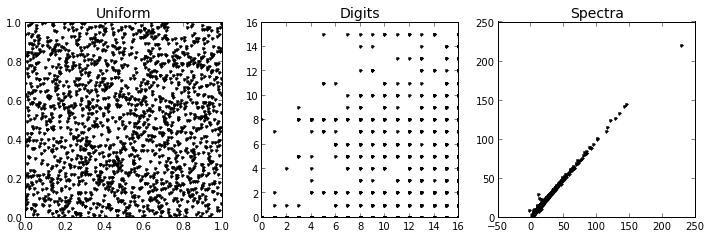We can see how different the structure is between these three sets. The uniform data is randomly and densely distributed throughout the space. The digits data actually comprise discrete values between 0 and 16, and more-or-less fill certain regions of the parameter space. The spectra display strongly-correlated values, such that they occupy a very small fraction of the total parameter volume.

### Benchmarking Scripts¶

Now we'll create some scripts that will help us to run the benchmarks. Don't worry about these details for now -- you can simply scroll down past these and get to the plots.

In :
from time import time

def average_time(executable, *args, **kwargs):
"""Compute the average time over N runs"""
N = 5
t = 0
for i in range(N):
t0 = time()
res = executable(*args, **kwargs)
t1 = time()
t += (t1 - t0)
return res, t * 1. / N

In :
TREE_DICT = dict(cKDTree=cKDTree, KDTree=KDTree, BallTree=BallTree)
colors = dict(cKDTree='black', KDTree='red', BallTree='blue', brute='gray', gaussian_kde='black')

def bench_knn_query(tree_name, X, N, D, leaf_size, k,
build_args=None, query_args=None):
"""Run benchmarks for the k-nearest neighbors query"""
Tree = TREE_DICT[tree_name]

if build_args is None:
build_args = {}

if query_args is None:
query_args = {}

NDLk = np.broadcast(N, D, leaf_size, k)

t_build = np.zeros(NDLk.size)
t_query = np.zeros(NDLk.size)

for i, (N, D, leaf_size, k) in enumerate(NDLk):
XND = X[:N, :D]

if tree_name == 'cKDTree':
build_args['leafsize'] = leaf_size
else:
build_args['leaf_size'] = leaf_size

tree, t_build[i] = average_time(Tree, XND, **build_args)
res, t_query[i] = average_time(tree.query, XND, k, **query_args)

return t_build, t_query

In :
def plot_scaling(data, estimate_brute=False, suptitle='', **kwargs):
"""Plot the scaling comparisons for different tree types"""
# Find the iterable key
iterables = [key for (key, val) in kwargs.iteritems() if hasattr(val, '__len__')]
if len(iterables) != 1:
raise ValueError("A single iterable argument must be specified")
x_key = iterables
x = kwargs[x_key]

# Set some defaults
if 'N' not in kwargs:
kwargs['N'] = data.shape
if 'D' not in kwargs:
kwargs['D'] = data.shape
if 'leaf_size' not in kwargs:
kwargs['leaf_size'] = 15
if 'k' not in kwargs:
kwargs['k'] = 5

fig, ax = plt.subplots(1, 2, figsize=(10, 4),
subplot_kw=dict(yscale='log', xscale='log'))

for tree_name in ['cKDTree', 'KDTree', 'BallTree']:
t_build, t_query = bench_knn_query(tree_name, data, **kwargs)
ax.plot(x, t_build, color=colors[tree_name], label=tree_name)
ax.plot(x, t_query, color=colors[tree_name], label=tree_name)

if tree_name != 'cKDTree':
t_build, t_query = bench_knn_query(tree_name, data,
**kwargs)
ax.plot(x, t_build, color=colors[tree_name], linestyle='--')
ax.plot(x, t_query, color=colors[tree_name], linestyle='--')

if estimate_brute:
Nmin = np.min(kwargs['N'])
Dmin = np.min(kwargs['D'])
kmin = np.min(kwargs['k'])

# get a baseline brute force time by setting the leaf size large,
# ensuring a brute force calculation over the data
_, t0 = bench_knn_query('KDTree', data, N=Nmin, D=Dmin, leaf_size=2 * Nmin, k=kmin)

# use the theoretical scaling: O[N^2 D]
if x_key == 'N':
exponent = 2
elif x_key == 'D':
exponent = 1
else:
exponent = 0

t_brute = t0 * (np.array(x, dtype=float) / np.min(x)) ** exponent
ax.plot(x, t_brute, color=colors['brute'], label='brute force (est.)')

for axi in ax:
axi.grid(True)
axi.set_xlabel(x_key)
axi.set_ylabel('time (s)')
axi.legend(loc='upper left')
axi.set_xlim(np.min(x), np.max(x))

info_str = ', '.join([key + '={' + key + '}' for key in ['N', 'D', 'k'] if key != x_key])
ax.set_title('Tree Build Time ({0})'.format(info_str.format(**kwargs)))
ax.set_title('Tree Query Time ({0})'.format(info_str.format(**kwargs)))

if suptitle:
fig.suptitle(suptitle, size=16)

return fig, ax


## Benchmark Plots¶

Now that all the code is in place, we can run the benchmarks. For all the plots, we'll show the build time and query time side-by-side. Note the scales on the graphs below: overall, the build times are usually a factor of 10-100 faster than the query times, so the differences in build times are rarely worth worrying about.

A note about legends: we'll show single-tree approaches as a solid line, and we'll show dual-tree approaches as dashed lines. In addition, where it's relevant, we'll estimate the brute force scaling for ease of comparison.

### Scaling with Leaf Size¶

We will start by exploring the scaling with the leaf_size parameter: recall that the leaf size controls the minimum number of points in a given node, and effectively adjusts the tradeoff between the cost of node traversal and the cost of a brute-force distance estimate.

In :
leaf_size = 2 ** np.arange(10)
for title, dataset in zip(titles, datasets_N):
fig, ax = plot_scaling(dataset, N=2000, leaf_size=leaf_size, suptitle=title)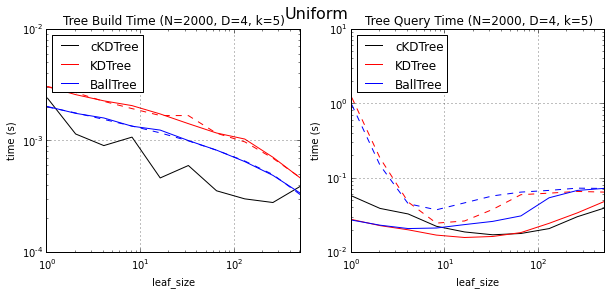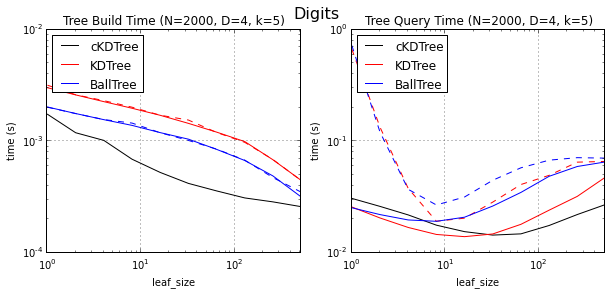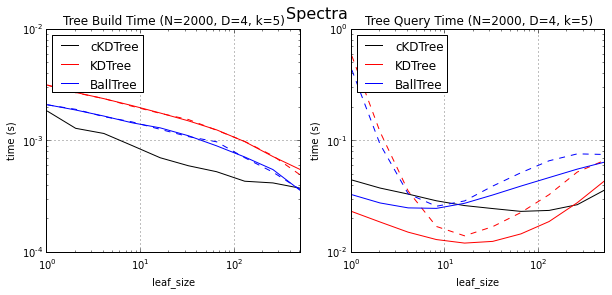Note that with larger leaf size, the build time decreases: this is because fewer nodes need to be built. For the query times, we see a distinct minimum. For very small leaf sizes, the query slows down because the algorithm must access many nodes to complete the query. For very large leaf sizes, the query slows down because there are too many pairwise distance computations. If we were to use a less efficient metric function, the balance between these would change and a larger leaf size would be warranted. This benchmark motivates our setting the leaf size to 15 for the remaining tests.

### Scaling with Number of Neighbors¶

Here we'll plot the scaling with the number of neighbors $k$. This should not effect the build time, because $k$ does not enter there. It will, however, affect the query time:

In :
k = 2 ** np.arange(1, 10)
for title, dataset in zip(titles, datasets_N):
fig, ax = plot_scaling(dataset, N=4000, k=k, suptitle=title,
estimate_brute=True)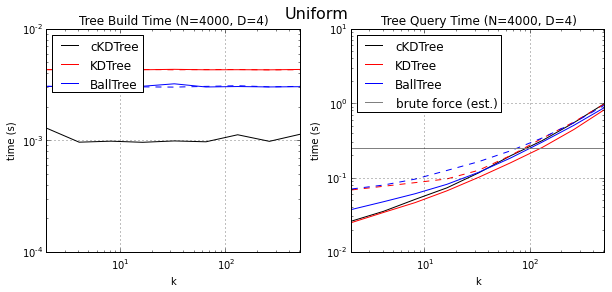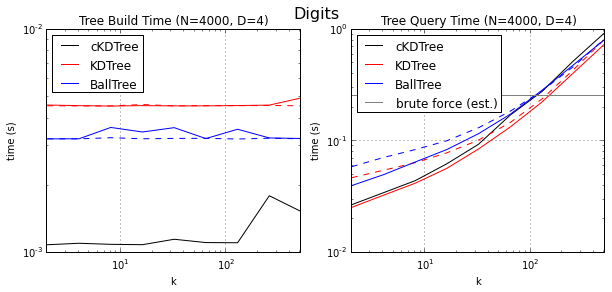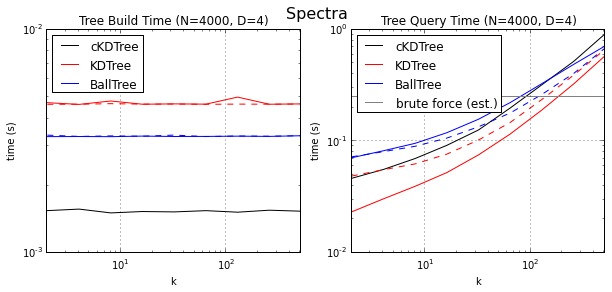Naively you might expect linear scaling with $k$, but for large $k$ that is not the case. Because a priority queue of the nearest neighbors must be maintained, the scaling is super-linear for large $k$.

We also see that brute force has no dependence on $k$ (all distances must be computed in any case). This means that if $k$ is very large, a brute force approach will win out (though the exact value for which this is true depends on $N$, $D$, the structure of the data, and all the other factors mentioned above).

Note that although the cKDTree build time is a factor of ~3 faster than the others, the absolute time difference is less than two milliseconds: a difference which is orders of magnitude smaller than the query time. This is due to the shortcut mentioned above: the cKDTree doesn't take the time to shrink the bounds of each node.

### Scaling with the Number of Points¶

This is where things get interesting: the scaling with the number of points $N$ :

In :
N = (10 ** np.linspace(2, 4, 10)).astype(int)
for title, dataset in zip(titles, datasets_N):
plot_scaling(dataset, N=N, estimate_brute=True, suptitle=title)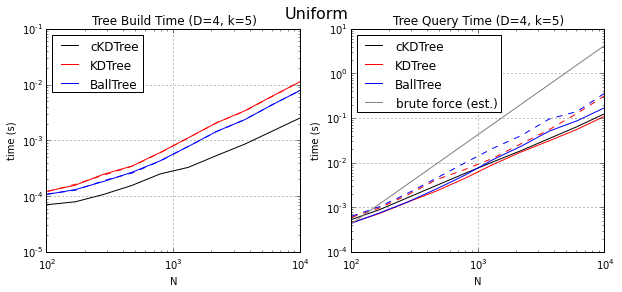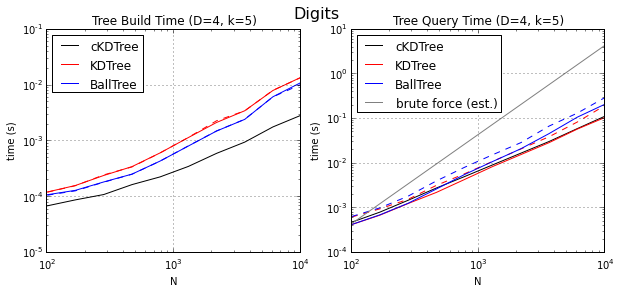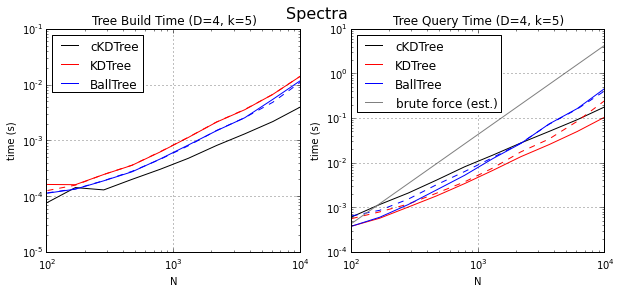We have set d = 4 and k = 5 in each case for ease of comparison. Examining the graphs, we see some common traits: all the tree algorithms seem to be scaling as approximately $\mathcal{O}[N\log N]$, and both kd-trees are beating the ball tree. Somewhat surprisingly, the dual tree approaches are slower than the single-tree approaches. For 10,000 points, the speedup over brute force is around a factor of 50, and this speedup will get larger as $N$ further increases.

Additionally, the comparison of datasets is interesting. Even for this low dimensionality, the tree methods tend to be slightly faster for structured data than for uniform data. Surprisingly, the cKDTree performance gets worse for highly structured data. I believe this is due to the use of the sliding midpoint rule: it works well for evenly distributed data, but for highly structured data can lead to situations where there are many very sparsely-populated nodes.

### Scaling with the Dimension¶

As a final benchmark, we'll plot the scaling with dimension.

In :
D = 2 ** np.arange(8)
for title, dataset in zip(titles, datasets_D):
plot_scaling(dataset, D=D, estimate_brute=True, suptitle=title)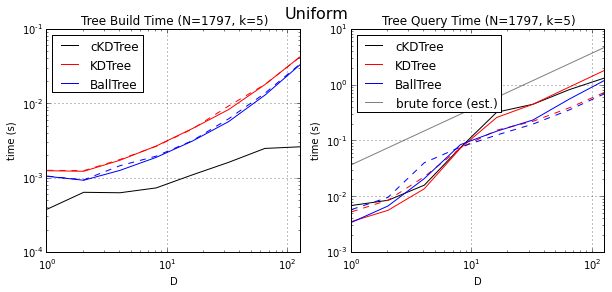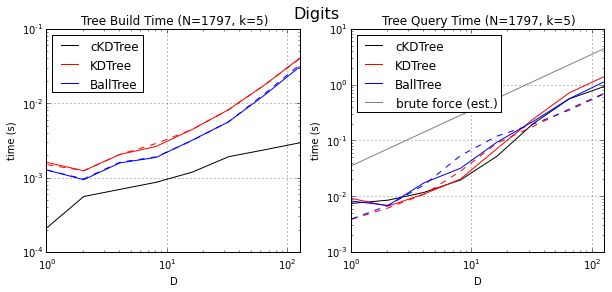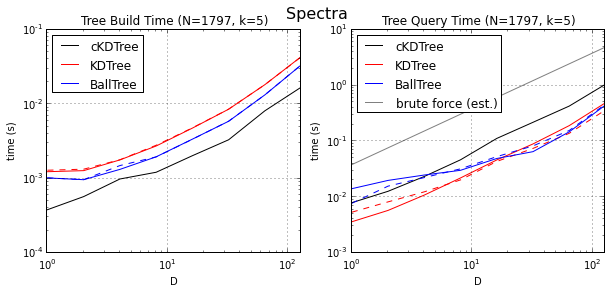As we increase the dimension, we see something interesting. For more broadly-distributed data (uniform and digits), the dual-tree approach begins to out-perform the single-tree approach, by as much as a factor of 2. In bottom-right panel, we again see a strong effect of the cKDTree's shortcut in construction: because it builds nodes which span the entire volume of parameter space, most of these nodes are quite empty, especially as the dimension is increased. This leads to queries which are quite a bit slower for sparse data in high dimensions, and overwhelms by a factor of 100 any computational savings at construction.

## Conclusion¶

In a lot of ways, the plots here are their own conclusion. But in general, this exercise convinces me that the new Ball Tree and KD Tree in scikit-learn are at the very least equal to the scipy implementation, and in some cases much better:

• All three trees scale in the expected way with the number and dimension of the data
• All three trees beat brute force by orders of magnitude in all but the most extreme circumstances.
• The cKDTree seems to be less optimal for highly-structured data, which is the kind of data that is generally of interest.
• The cKDTree has the further disadvantage of using dynamically allocated nodes, which cannot be serialized. The pre-allocation of memory for the new ball tree and kd tree solves this problem.

On top of this, the new ball tree and kd tree have several other advantages, including more flexibility in traversal methods, more available metrics, and more availale query types (e.g. KDE and 2-point correlation).

One thing that still puzzles me is the fact that the dual tree approaches don't offer much of an improvement over single tree. The literature on the subject would make me expect otherwise (FastLab, for example, quotes near-linear-time queries for dual tree approaches), so perhaps there's some efficiency I've missed.

In a later post, I plan to go into more detail and explore and benchmark some of the new functionalities added: the kernel density estimation and 2-point correlation function methods. Until then, I hope you've found this post interesting, and I hope you find this new code useful!

This post was written entirely in the IPython notebook. You can download this notebook, or see a static view here.